Steam Paathshaala Economics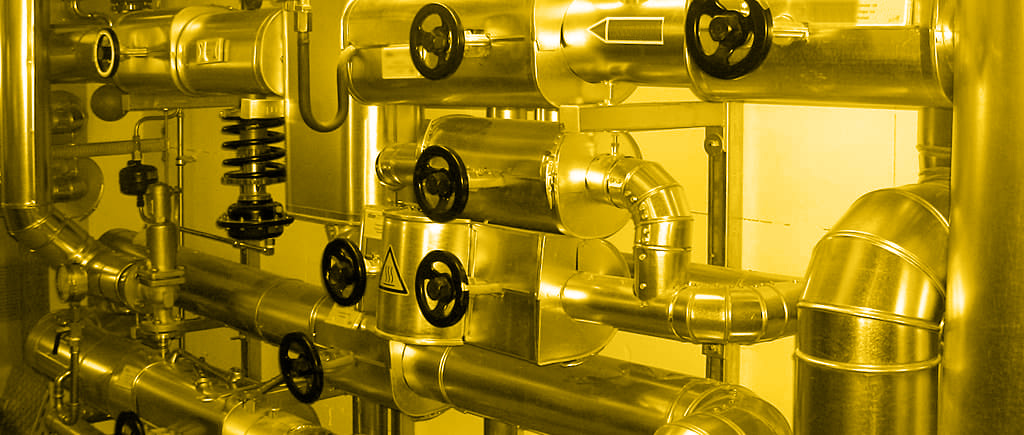# Economics

## Heat saving calculation

Let us first understand how costs / savings are calculated. We know that,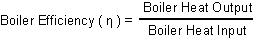Boiler Heat Input is the energy from fuel, and boiler Heat Output is the energy in steam.

Boiler Heat Input = Qf x GCV

where,
Qf = Quantity of fuel (in kg/hr)
GCV (Gross Calorific Value) =Energy contained in fuel in kcal/kg

Boiler Heat Output = Qs x (Hs - Hw)

where,
Qs = Quantity of steam (in kg/hr)
Hs = Heat contained in steam (Enthalpy of Saturated steam hg)
Hw = Heat already present in the water from which steam is raised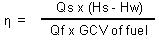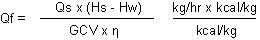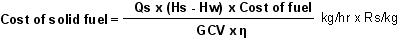To find out the fuel cost for liquid/gas fuels, we need to divide by the Specific gravity (ρ) of that fluid.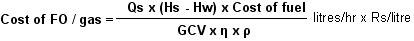This is the basic equation we will work with for allocating fuel cost.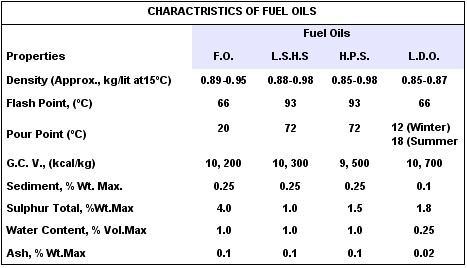## Cost of steam

Example 5.1.
Let us take a 5TPH (tons per hour) oil-fired boiler, @10.5 kg/cm2g, using DM water at an ambient temperature of 30°C.

Direct cost
First, we calculate the Direct costs of
• Fuel
• Water
• Water treatment
• Electricity

Fuel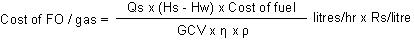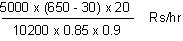= 7945.66 Rs/hr

Assume the boiler operates for 24 hours a day for 335 days a year which is about 8000 hours per year. So,
Cost of FO = 7945.66 x 8000 Rs/year = Rs. 635.6 lakhs/ year (A)

Water
A 5TPH boiler will use 5 tons of water per hour. Assume water cost as Rs. 10/klitre = Rs. 10/ ton of water. So, Cost of water = 5 x 10 x 8000 = Rs. 4 lakhs/year (B)

Water treatment
Assume DM water treatment cost as Rs. 30/Klitre. So,

Cost of treatment = 5 x 30 x 8000 = Rs. 12 Lakhs/year (C)

Electricity
Electricity is used by every boiler for running its feedpumps, blowers, controls, etc. assume electricity consumption @ 40 Kw-hr. Also, take the cost of electricity at Rs. 4.50p per Kw-hr. So,

Cost of electricity = 40 x 4.5 x 8000 = Rs. 14.4 lakhs/year (D)

Indirect costs
These are the costs of
• Manpower
• Capital cost of -Space, Boiler cost, Depreciation

Manpower
Assume 3 IBR boiler Operators (salary Rs. 8000/year) and one boiler Supervisor (salary Rs. 10,000/year).
Total cost = Rs. 4.1 lakhs/year (E)

Capital cost
We will take out the cost of finance of both the space and the boiler.
Space cost. 5000 sq. ft of boilerhouse space x Rs. 400/sq.ft. = Rs. 20 lakhs.
Boiler cost. Rs. 50 lakhs

Finance cost of Rs. 70 lakhs = 0.15 x 70 = Rs. 10 lakhs/year
Depreciation of boiler = 0.15 x 50 = 7.5 lakhs/year

A total Capital cost = Rs. 17.5 lakhs/year (F)

Adding A + B + C + D + E + F, we get Rs. 687.6 lakhs for a 5 TPH boiler/year.

Or, Rs. 137 lakhs / ton / year, or, Rs. 1,719 / TPH !!

## Cost of leaks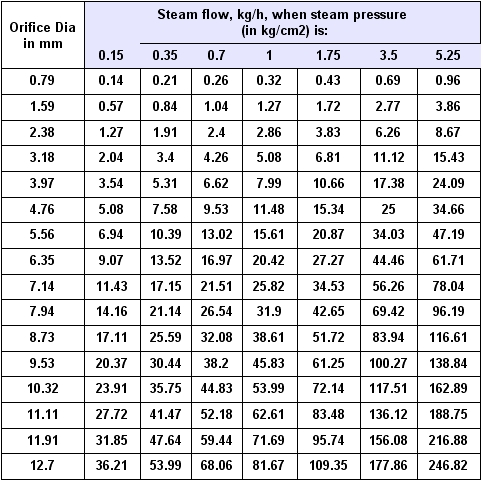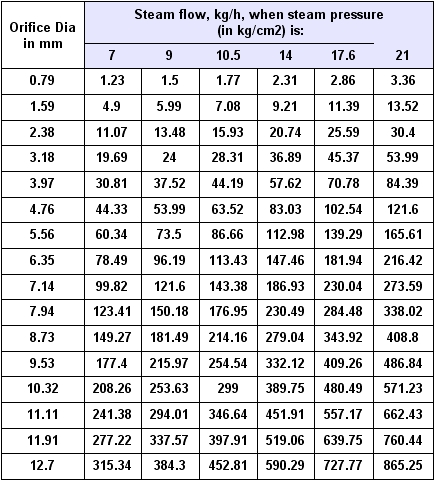## Cost of Condensate

In the boiler , we heat water by suppling it energy in the form of heat. When the temperature goes above boiling point of water it changes state & converts to steam. Steam is the form in which this heat energy is now carried to the process. Lets say a jacketed vessel containing some liquid which needs to be heated. When steam is supplied to this cooler material to be heated, the energy is transferred from steam which new changes back to liquid state- i.e it condenses. This hot water is called condensate.

Unfortunately, in practice not all of this heat energy is transferred. Only 75% of the total energy carried by steam is transferred. What happens to the rest? That”s right. It is now in the condensate. So, if the total energy produced by the boiler is 100, units only 75 is transferred to the process. 25 units is trapped in the condensate.

This condensate collects in the pipes which are at a high pressure. When condensate is drained out from steam trap to a lower pressure, instantaneously some of the condensate re-evaporates as Flash Steam. About half of the energy carried by the condensate can be cost in this way. So ,out of the 25 units of heat in condensate 12.5 units just evaporates.

What is Condensate Management?
We have already explained condensate and flash steam. When we talk of removing condensate, recovering it and receiving flash steam as well, this is called Condensate Management

It is easy to figure that collecting & recovering both condensate & flash steam is very critical not just in money terms but also to prevent wastage.

How Much can Condensate recovery save the client?

• MoneySaving : Of fuel

• MoneySaved : Of water Conserved

• Money Saved : Of chemicals

Condensate return cuts cost of water
Condensate is after all water, and when returned to the boiler slashes water bills from the government, or, reduces electricity costs if we are pumping it up from a nearby river.

Condensate is ideal Boiler feedwater
As condensate comes from pure steam it is distilled water with no dissolved solids. ( very low TDS). If condensate is returned, the boiler needs lesser blowdown which is a big economic consideration as the boiler loses energy each time it blows down.

For blowdown and TDS talk see Chemistry / TDS section

Not just that, because we are recycling condensate, we save the cost of chemical treatments that we would have to do, were we using fresh make-up water.

When cold water is used to top up the boiler, naturally the boilers efficiency is compromised the lower the feed water temperature, the lesser the steam a boiler produces.

The boiler´s “From and At rating” falls. Cold water put into the boiler might even lead to thermal shock.

Condensate saves chemical costs
As condensate is pure water, and it has already been treated, it can be used as is in the boiler. This cuts the cost of water treatment, too.

How do we determine how much condensate recovery saves the client in monetary terms? Simple. Condensate returned, means fuel saved. How much this fuel saving is, can be determined from the formula: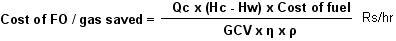where,
Qc = Quantity of condensate (in kg/hr)
Hc = Heat contained in condensate (in kcal/kg)
Hw = Heat already present in the water at ambient tenmperature (in kcal/kg)

GCV (Gross Calorific Value) =Energy contained in fuel in kcal/kg
η = Boiler efficiency
ρ = Specific gravity of liquid/gas fuel

Example 5.2. Find the cost savings from one ton of condensate at 100°C, 0 kg/cm2g on an oil-fired boiler.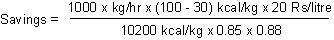= Rs. 183.5/ hour x 800 hrs = Rs. 14.68 lakhs/ year

There is also the added savings from water and water treatment which is calculated similar to Example 5.1.

## Cost of Flash

If sufficiently hot condensate from a pressurized system is released to a lower pressure, some of that condensate will have the heat necessary to become steam. This is called flash steam.

‘Flash steam’ is released from hot condensate when its pressure is reduced. Even water at room temperature of 35°C would boil if its pressure were lowered far enough. It may be worth noting that water at 170°C will boil at any pressure below 7 kg/cm2g. The steam released by the flashing process is as useful as steam released from a steam boiler.

Example 5.3.
What is the total savings from 1 TPH condensate @ 7barg on oil?
First we use a flash separator to separate out flash at 0.5 barg.
The quantity of flash separated can be found from the formula: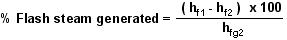Where,
hf1 = Enthalpy of water at the higher pressure kcal/kg
hf2 = Enthalpy of water at the flashing pressure kcal/kg
hfg2 = Enthalpy of evaporation at the flash steam pressure

% of flash steam generated = hf (7barg) - hf (0.5 barg) / hfg (0.5 barg) = 172 - 111/ 532 x 100 = 11.5 %
The above formula, in fact, is used to make the graph below.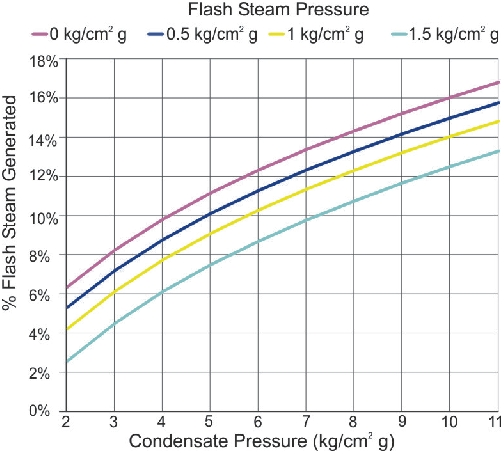Quantity of flash is therefore, 11 % of 1000 kg/hr = 111 kg/hr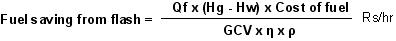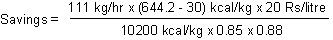= Rs 178.7/hour x 8000 hrs = Rs. 14.29 lakhs/year

After removing flash, we will also recover condensate. The quantity of condensate available is
= (1000 - 111 ) kg/hr = 889 kg/hr

The savings from this can be found out similar to Example 5.2. It comes to Rs. 13.05 lakhs/year.The savings from flash in most cases is as much, if not more than condensate.